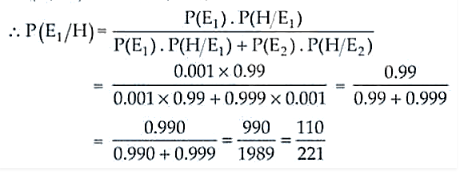# By examining the chest X ray,

Question:

By examining the chest X ray, the probability that TB is detected when a person is actually suffering is 0.99. The probability of an healthy person diagnosed to

have TB is 0.001. In a certain city, 1 in 1000 people suffers from TB. A person is selected at random and is diagnosed to have TB. What is the probability that he

actually has TB?

Solution:

Let E1 = Event that a person has TB

E2 = Event that a person does not have TB

And H = Event that the person is diagnosed to have TB.

So,

P(E1) = 1/1000 = 0.001, P(E2) = 1 – 1/1000 = 999/1000 = 0.999

P(H/E1) = 0.99, P(H/E2) = 0.001

Now, using Baye’s theorem we haveTherefore, the required probability is 110/221.